# Generalized Lucas numbers of the form 3 × 2m

Salah Eddine Rihane, Chefiath Awero Adegbindin and Alain Togbé
Notes on Number Theory and Discrete Mathematics
Print ISSN 1310–5132, Online ISSN 2367–8275
Volume 27, 2021, Number 2, Pages 129—136
DOI: 10.7546/nntdm.2021.27.2.129-136

## Details

### Authors and affiliations

Salah Eddine RihaneDepartment of Mathematics and Computer Science
Abdelhafid Boussouf University
Mila 43000, Algeria

Chefiath Awero AdegbindinInstitut de Mathematiques et de Sciences Physiques
Dangbo, Benin

Alain TogbéDepartment of Mathematics, Statistics, and Computer Science
Purdue University Northwest
1401 S, U.S. 421, Westville IN 46391, United States

### Abstract

For an integer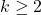, letbe the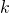-generalized Lucas sequence which starts with(terms) and each term afterwards is the sum of thepreceding terms. In this paper, we look the-generalized Lucas numbers of the form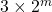i.e. we study the Diophantine equation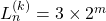in positive integers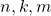with.

### Keywords

• k-generalized Lucas numbers
• Linear form in logarithms
• Reduction method

• 11B39
• 11J86

### References

1. Adegbindin, C., Luca, F., & Togbé, A. (2019). Lucas numbers as sums of two repdigits. Lithuanian Mathematical Journal, 59(3), 295–304.
2. Baker, A., & Davenport, H. (1969). The equations 3x2 − 2 = y 2 and 8x2 − 7 = z2. Quarterly Journal of Mathematics, Oxford Series (2), 20, 129–137.
3. Bravo, J. J., & Luca, F. (2012). Powers of two in generalized Fibonacci sequences. Revista Colombiana de Matemáticas, 46, 67–79.
4. Bravo, J. J., Gómez, C. A., & Luca, F. (2016). Powers of two as sums of two k-Fibonacci numbers. Miskolc Mathematical Notes, 17, 85–100.
5. Bravo, J. J., & Luca, F. (2014). Repdigits in k-Lucas sequences. Proceedings of the Indian Academy of Sciences: Mathematical Sciences, 124(2), 141–154.
6. Dujella, A., & Pethő, A. (1998). A generalization of a theorem of Baker and Davenport. Quarterly Journal of Mathematics, Oxford Series (2), 49(195), 291–306.
7. Matveev, E. M. (2000). An explicit lower bound for a homogeneous rational linear form in the logarithms of algebraic numbers, II. Izvestiya: Mathematics, 64(6), 1217–1269.
8. Mile, E. P. Jr. (1960). Generalized Fibonacci numbers and associated matrices. American Mathematical Monthly, 67, 745–752.
9. Miller, M. D. (1971). Mathematical notes: on generalized Fibonacci number. American Mathematical Monthly, 78, 1108–1109.
10. Pethő, A. (1982). Perfect powers in second order linear recurrences. Journal of Number Theory , 15, 5–13.
11. Rihane, S. E., Faye, B., Luca, F., & Togbé, A. (2020). Powers of two in generalized Lucas sequences. The Fibonacci Quarterly, 58(3), 254–260.
12. Wolfram, D. A. (1998). Solving generalized Fibonacci recurrences. The Fibonacci Quarterly, 36(2), 129–145.

## Cite this paper

Rihane, S. E., Adegbindin, C. A., & Togbé, A. (2021). Generalized Lucas numbers of the form 3 × 2m. Notes on Number Theory and Discrete Mathematics, 27(2), 129-136, doi: 10.7546/nntdm.2021.27.2.129-136.